# Lebesgue constants of multi-dimensional partial Fourier sums

(diff) ← Older revision | Latest revision (diff) | Newer revision → (diff)

Letbe an integrable function on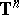,,,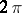-periodic in each variable. Consider its Fourier series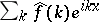, where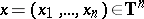,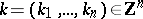, the lattice of points inwith integer coordinates,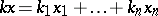, while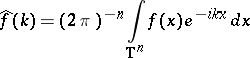is theth Fourier coefficient of. No natural ordering of Fourier coefficients exists, thus the definition of a multi-dimensional partial Fourier sum presents many problems and points of interest intimately connected to geometry and number theory.

To indicate that the partial sum corresponds to a certain summation domain, one denotes it byFrequently, sumsare considered, where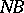is theth dilatation of a fixed set; in many cases this is the most natural way of summation. An example of partial Fourier sums that are not of this kind are the rectangular partial sums. Byone denotes the partial Fourier sum when the dependence on the parameter, either scalar or vectorial, is of primary importance. As is well-known, if the Fourier series of a continuous function fails to converge at each point, then the sequence of norms of the operators,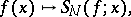taking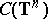into(or, equivalently,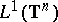into) is unbounded and measures the rate of divergence of the Fourier series.

This is strongly related to the behaviour of the Fourier transform of the indicator function of the summation domain. For known results on this subject, see, e.g., [a11], [a13], [a18].

For the spherical partial Fourier sums, where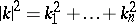, the following order estimate holds: There exist positive constants, and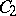,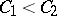, depending only on, such that(a1)

The estimate from below has first appeared in [a14]; the method used there is the main tool for obtaining lower bounds for Lebesgue constants. Nothing is known about existence or non-existence of the limit of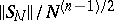as; this is the main open problem in the subject (as of 2000).

More general summation domainspossessing properties of the spherical partial Fourier sums have been considered. E.g., in Yudin's estimate from above [a25], the summation domainsare balanced (i.e., along with each pointthe whole set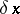,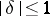, belongs to), with finite upper Minkowski measure, that is,where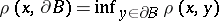. These natural assumptions provide the bound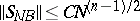. It turns out that to satisfy the same estimate from below, only local information is needed ([a17]): Let the boundary of a domaincontain a simple (non-intersecting) piece of a surface of smoothness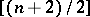in which there is at least one point with non-vanishing principal curvatures (cf. also Principal curvature). Then there exists a positive constant, depending only on, such that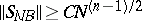forlarge.

The estimates in the spherical case and its generalizations are the worst possible ifis compact. Oncehas a point with non-vanishing principal curvatures, the Lebesgue constants are that "bad" . The other side of the scale is called "polyhedral" and is of "logarithmic nature" . Only some natural restrictions have to be put on polyhedra, for example, the hyperplanes that define the sides of the polyhedron do not contain the origin. In that case there exist two positive constants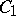and,, such that for each such polyhedron:(a2)

Actually, this was proved by E. Belinsky [a6].

There are two important problems concerning the polyhedral case. The first is: Can partial Fourier sums have Lebesgue constants with an intermediate rate of growth (i.e. between (a1) and (a2))? Some trivial solutions were suggested in [a26], where an intermediate growth is achieved by the product of the two mentioned situations. Of course, this is possible only for dimension three and greater. Thus, the problem is to find one for dimension two. It is clear that in this case the boundary can possess no point with non-vanishing curvature. On the other hand, any polyhedron matches (a2). Thus, the solution can only be a (convex) "polyhedron" with infinitely many specially located sides. Such a solution was constructed by A. Podkorytov [a21].

The next question also seems very natural: Is it possible to have a certain asymptotic relation instead of the order estimate (a2)? For rectangular partial sums some special cases were investigated by I. Daugavet [a9] and O. Kuznetsova [a15]. For the sequence of dilated summation domains, an unexpected result was obtained by Podkorytov [a22]. Herealso causes the main difficulties. There are two main cases. In the first one, the polygonswith sides of rational slopes are dealt with — then the estimates change insignificantly if only one considers instead of sums the corresponding integrals, that is, the Fourier transformof the indicator function of the-dilation of the corresponding set. This allows one to obtain logarithmic asymptotics; namely, the values,and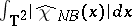are equivalent. When at least one of the slopes is irrational, the situation changes qualitatively: The upper limit and the lower limit of the ratio, as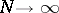, may differ. In [a22] and [a20], quantitative estimates of this phenomenon as well as open problems are given.

The paper [a4] started the interest in various questions of approximation theory and Fourier analysis inconnected with the study of hyperbolic cross partial Fourier sums (see, e.g., [a24] and Hyperbolic cross). The exact order of growth of their Lebesgue constants,, the same as in the spherical case, was established in the two-dimensional case independently in [a5] and in [a27], and afterwards was generalized to the case of arbitrary dimension in [a16].

Step hyperbolic crosseswere introduced by B. Mityagin [a19] and are defined as follows (cf. also Step hyperbolic cross):forsuch that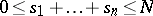. These have many important applications too. Belinsky [a8] proved that there exist two positive constantsand,, such that.

Whenis unbounded, it may happen that the operator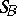is unbounded even for fixed. It is proved in [a3] that the Lebesgue constants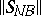are either of the usual order of growth or infinite for all values of the parameter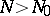, whereis a hyperbolic cross, depending on whether the hyperbolic cross is turned at a rational or irrational angle, respectively. For, this was earlier obtained in [a7], which also contains similar results for the strip to be a summation domain.

For other results on Lebesgue constants and related topics, see [a1], [a2], [a10], [a12], [a18], [a23], [a28], [a29].

The ideas used to prove many of the results discussed above have also been applied to estimates of the Lebesgue constants of linear means of multiple Fourier series.

Some results are known for Lebesgue constants in more abstract settings, e.g., for spherical harmonics expansions or Fourier series on compact Lie groups.

How to Cite This Entry:
Lebesgue constants of multi-dimensional partial Fourier sums. Encyclopedia of Mathematics. URL: http://encyclopediaofmath.org/index.php?title=Lebesgue_constants_of_multi-dimensional_partial_Fourier_sums&oldid=14045
This article was adapted from an original article by E.R. Liflyand (originator), which appeared in Encyclopedia of Mathematics - ISBN 1402006098. See original article